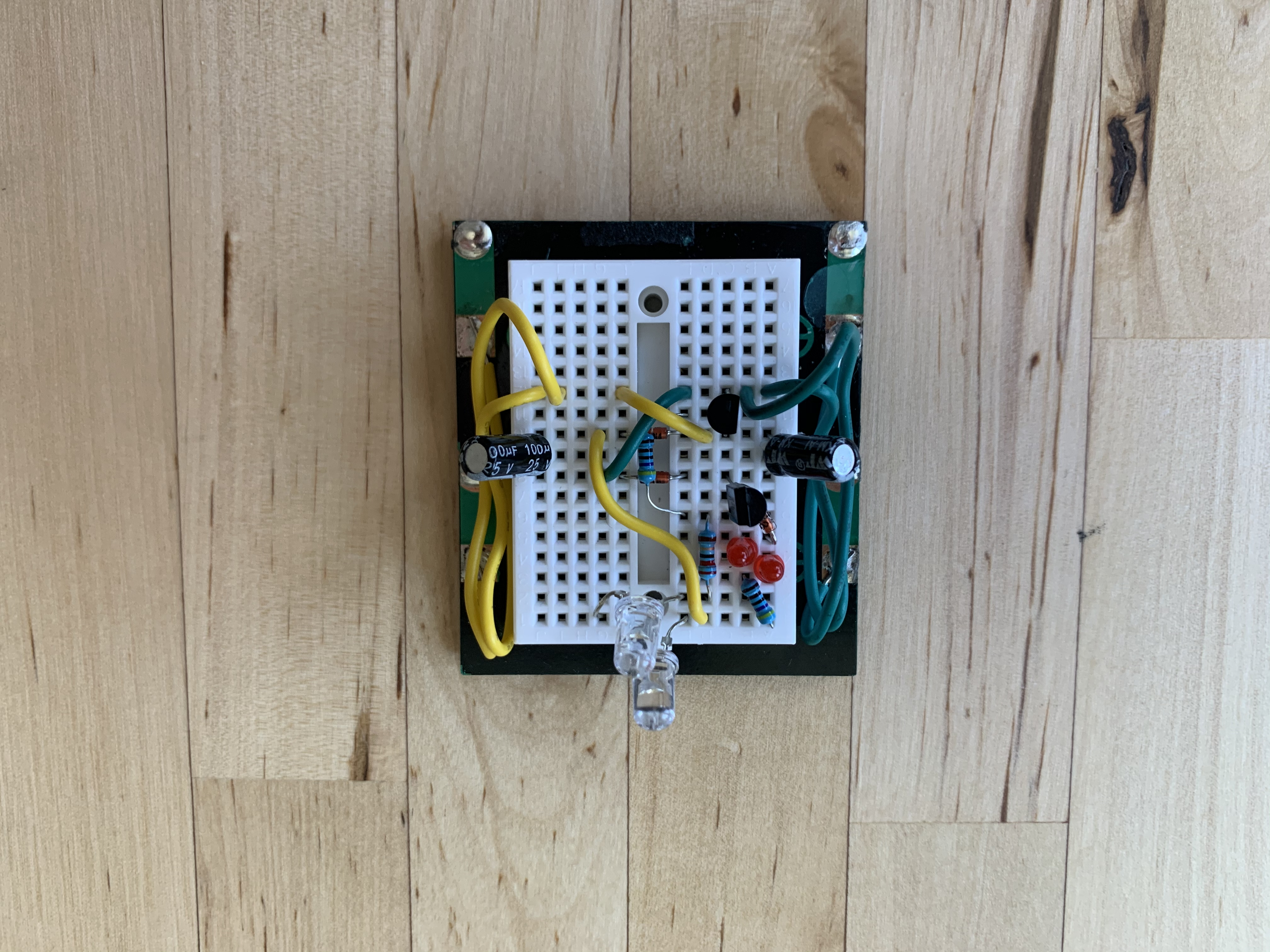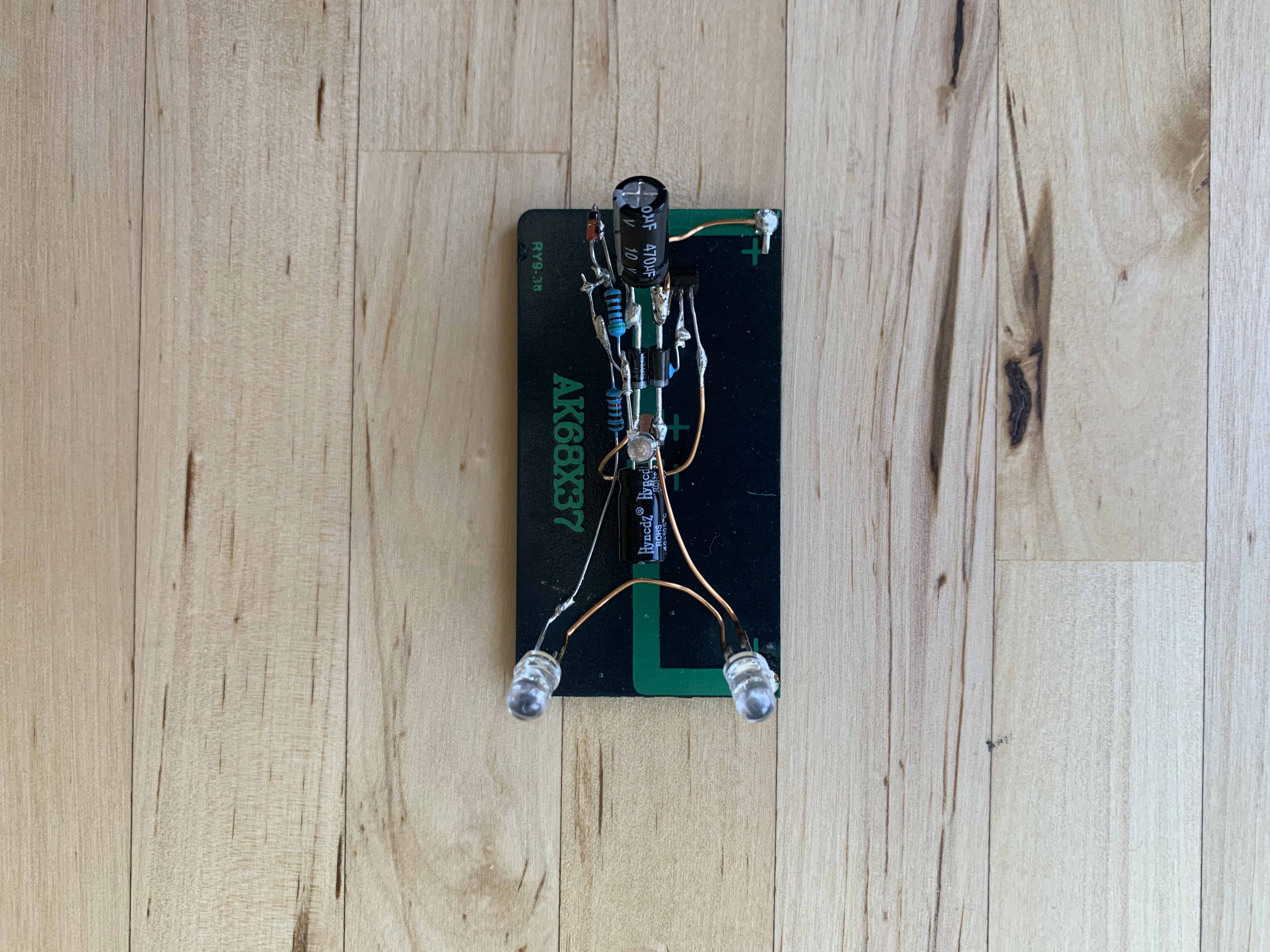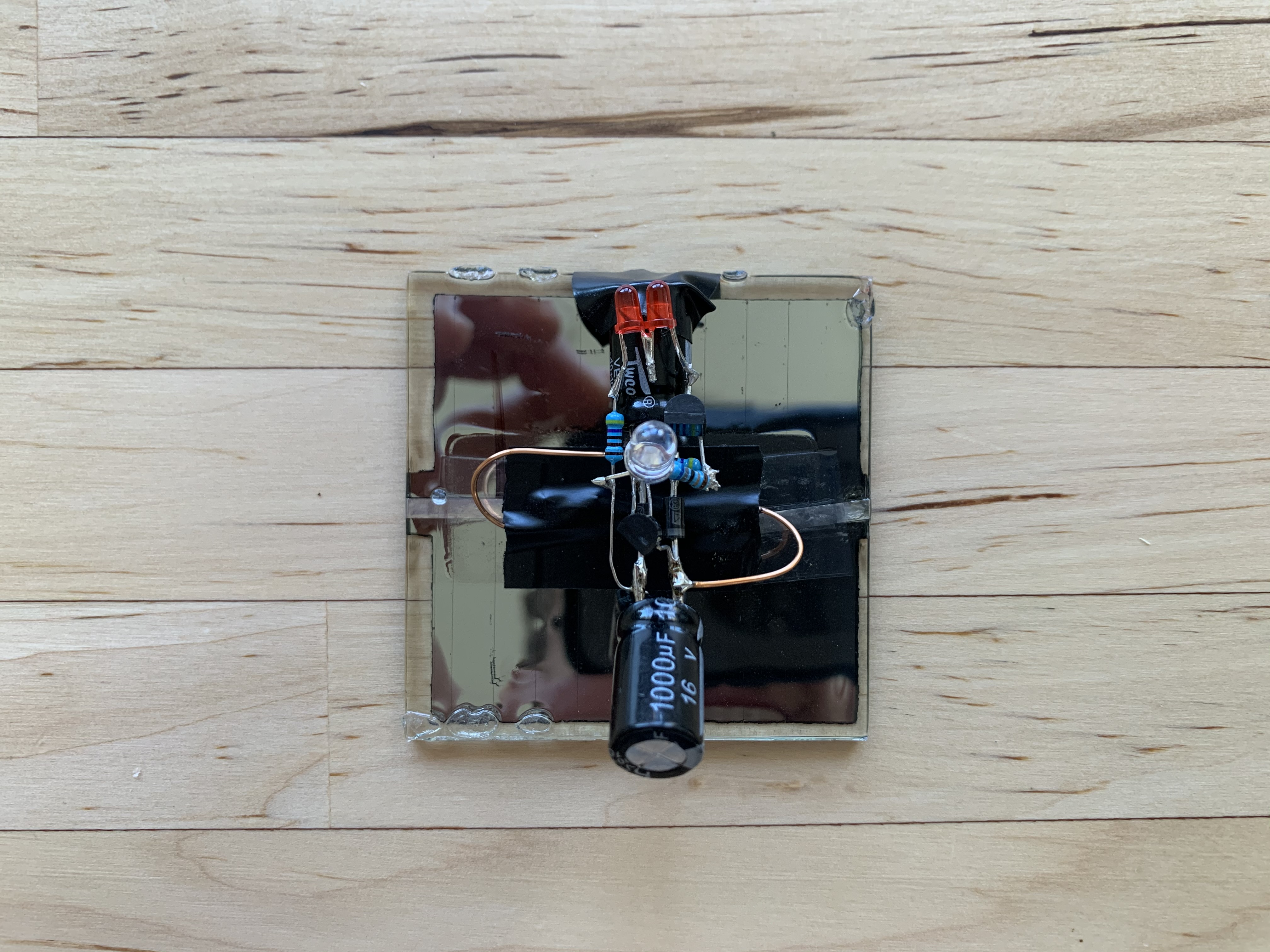# Julian Straub

## creations and thoughts

In a previous post about harvesting light with a charge pump, I described my first iteration on that classic circuit. Since them I have built a few more iterations and I have learned a couple of things.

## 6V Solar Harvester

First, I worked on a 6V version of the harvester since I got a lot of 6V solar cells. You can see the 6V version can power at least two green LEDs:

I adapted the parts of the original 4V blinker to:

• $$R_0=4.7k\Omega, R_1=4.7k\Omega, R_2=22\Omega, C_0=C_1=100\mu F$$ .
• $$D_0=D_1=$$ 1N4148.
• $$T_0=$$ 2N2222 and $$T_1=$$ 2N3906.
• $$D_2=$$ two low power red LEDs and a 1N4148 in series.
• $$D_3=$$ two ultra-bright green LEDs in series.
• two small 6V solar cells in parallel to double the power.From that I designed a free-formed version of the 6V blinkerSimilar parts as the 6V harvester but using a ultra-bright green LED in series with a 1N4148 diode for $$D_2$$ .

• $$R_0=5.1k\Omega, R_1=5.1k\Omega, R_2=120\Omega, C_0=C_1=470\mu F$$
• $$D_0=D_1=$$ Schottky
• $$T_0=$$ 2N2222 and $$T_1=$$ 2N2907
• $$D_2=$$ ultra-bright green LED and a 1N4148 in series for about 4V
• $$D_3=$$ two ultra-bright green LEDs in series
• a larger 6V solar cell.

The interesting effect is that the ultra-bright green LED that is really just meant to set the trigger voltage, is actually sensitive enough to light up in addition to the other two ultra-bright green LEDs as can be seen in the video below:

## 4V Solar HarvesterSimilar to the initial version this 4V solar harvester used:

• $$R_0=4.7k\Omega, R_1=4.7k\Omega, R_2=68\Omega, C_0=C_1=1mF$$ .
• $$D_0=D_1=$$ Schottky
• $$T_0=$$ 2N3904 and $$T_1=$$ 2N3906
• $$D_2=$$ two low power red LED
• $$D_3=$$ one ultra-bright green LED.
• 4V max - rated for 3V solar cell.

In experimenting with the initial version, I realized that using two red LEDs for $$D_2$$ pushes the trigger point higher but not too high for it to never trigger. The resulting flashes are way brighter. Satisfying to be able to perceive the green light flashes reflected from the wall across the room of the harvester ;).

## Transistor Types Matter

I used the 2N2907 pnp transistor for the first time and it seemed it does not work as well as the 2N3906. The brightness of the LEDs during blinking noticeably decreased. I verified that by swapping it in the breadboard 6V Harvester from above. Checking on the datasheets its pretty clear that the 2N2907 is geared more for higher power use cases than the 2N3906 (the current gain for example is specified at 10V for the 2N2907 and at 1V for the 2N3906).

It seems a similar relationship exists between 2N3904 and 2N2222.